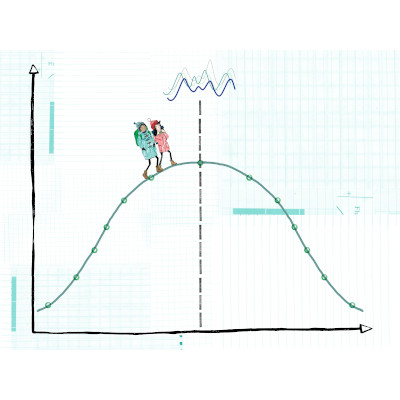GitHub

# Statistical Modelling with RCourse Level: Intermediate (6 hours)

From the very beginning, R was designed for statistical modelling. Out of the box, R makes standard statistical techniques easy. This course covers the fundamental modelling techniques. We begin the day by revising hypotheses tests, before moving onto ANOVA tables and regression analysis. The class ends by looking at more sophisticated methods such as clustering and principal components analysis (PCA).

Start Date:
Price:
Venue Details:
Time:
Duration:

## No Events Currently Scheduled

Sorry, there are no upcoming events for this course, but please get in touch if you would like to be kept informed when events are scheduled in the future.

## Course Details

### Outline

• Basic hypothesis testing: Examples include the one-sample t-test, one-sample Wilcoxon signed-rank test, independent two-sample t-test, Mann-Whitney test, two-sample t-test for paired samples, Wilcoxon signed-rank test.
• ANOVA tables: One-way and two-way tables.
• Simple and multiple linear regression: Including model diagnostics.
• Clustering: Hierarchical clustering, k-means.
• Principal components analysis: Plotting and scaling data.

### Learning Outcomes

By the end of the day participants will…

• have a thorough understanding of popular statistical techniques
• have the skills to make appropriate assumptions about the structure of the data and check the validity of these assumptions in R
• be able to fit regression models in R between a response variable understand how to apply said techniques to their own data using R’s common interface to statistical functions
• be able to cluster data using standard clustering techniques

### Prior Knowledge

It will be assumed that participants are familiar with R. For example, inputting data, basic visualisation and data frames. Attending the Introduction to R course will provide sufficient background. This course is suitable for all fields of work. Previous attendees include biologists, statisticians, accountants, engineers & students, i.e., anyone who uses a spreadsheet!

## Materials Preview

•••••